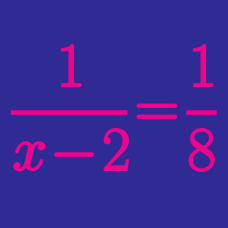Algebra

# Graphing Rational Equations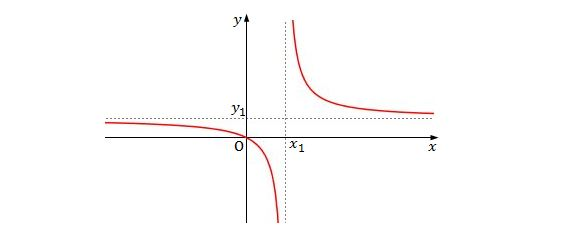The graph of the rational function $y=\frac{ax-b}{-2x+c}$ is as shown above. If $x_1=5$ and $y_1=4,$ what is the value of $a+b+c ?$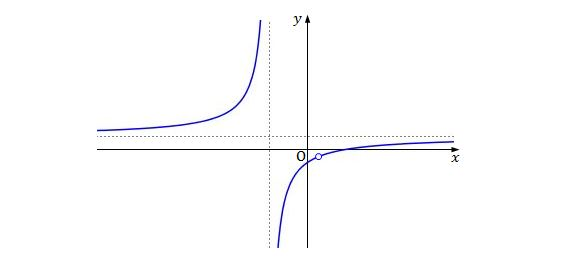Which of the following functions represents the above graph?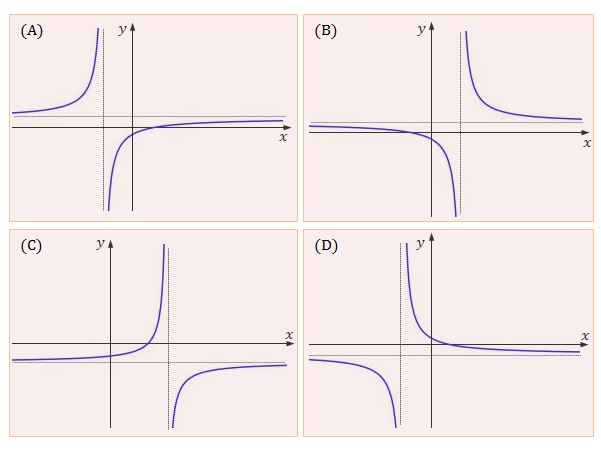Which of the above graphs is represented by the function $y=\frac{-x+9}{x-14} ?$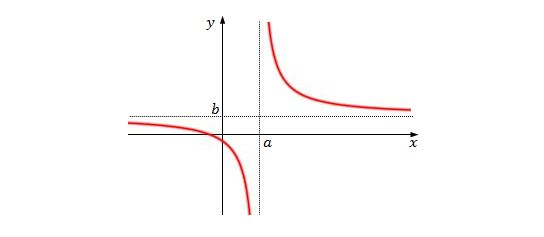Assuming that $a=6$ and $b=3,$ which of the following functions represents the above graph?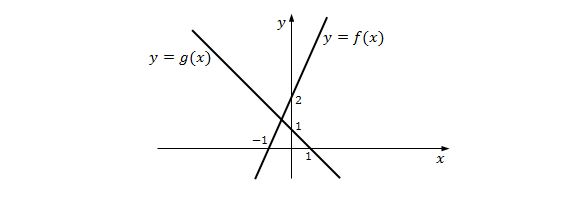If the graphs of two functions $y=f(x)$ and $y=g(x)$ are as shown above, which of the following is the graph of the function $\displaystyle y=\frac{f(x)}{g(x)}?$×